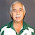## Monday, December 26, 2022

### Maximum number of points with minimum distance

In  the following problem is posted:

Consider $$N$$ points and a minimum distance $$\mathit{\color{darkblue}{MinDist}}$$. Pick as many points as possible from this collection, such that the distances between the selected points is at least $$\mathit{\color{darkblue}{MinDist}}$$.

A small data set can look like:

----      9 PARAMETER p  coordinates of points

x           y

i1       17.175      84.327
i2       55.038      30.114
i3       29.221      22.405
i4       34.983      85.627
i5        6.711      50.021
i6       99.812      57.873
i7       99.113      76.225
i8       13.069      63.972
i9       15.952      25.008
i10      66.893      43.536
i11      35.970      35.144
i12      13.149      15.010
i13      58.911      83.089
i14      23.082      66.573
i15      77.586      30.366
i16      11.049      50.238
i17      16.017      87.246
i18      26.511      28.581
i19      59.396      72.272
i20      62.825      46.380
i21      41.331      11.770
i22      31.421       4.655
i23      33.855      18.210
i24      64.573      56.075
i25      76.996      29.781
i26      66.111      75.582
i27      62.745      28.386
i28       8.642      10.251
i29      64.125      54.531
i30       3.152      79.236
i31       7.277      17.566
i32      52.563      75.021
i33      17.812       3.414
i34      58.513      62.123
i35      38.936      35.871
i36      24.303      24.642
i37      13.050      93.345
i38      37.994      78.340
i39      30.003      12.548
i40      74.887       6.923
i41      20.202       0.507
i42      26.961      49.985
i43      15.129      17.417
i44      33.064      31.691
i45      32.209      96.398
i46      99.360      36.990
i47      37.289      77.198
i48      39.668      91.310
i49      11.958      73.548
i50       5.542      57.630


One way to formulate this is using a quadratic model:

MIQCP Model
\begin{align}\max&\sum_i \color{darkred}x_i \\ & \mathit{\color{darkblue}{Dist}}_{i,j} \ge \mathit{\color{darkblue}{MinDist}} \cdot \color{darkred}x_i \cdot \color{darkred}x_j && \forall i\lt j \\ & \color{darkred}x_i \in \{0,1\}\end{align}

The quadratic constraint implement the implication $\color{darkred}x_i=\color{darkred}x_j=1 \Longrightarrow \mathit{\color{darkblue}{Dist}}_{i,j} \ge \mathit{\color{darkblue}{MinDist}}$ I.e., the constraint is not binding when either $$\color{darkred}x_i=0$$ or $$\color{darkred}x_j=0$$. Note that the input is just the distance matrix. We don't need to know the coordinates of the points. This means that this model works for any dimension (2d in this case or 3d in the original question).

We can easily linearize the model:

Linear MIP Model
\begin{align}\max&\sum_i \color{darkred}x_i \\ & \mathit{\color{darkblue}{Dist}}_{i,j} \ge \mathit{\color{darkblue}{MinDist}} \cdot ( \color{darkred}x_i + \color{darkred}x_j -1) && \forall i\lt j \\ & \color{darkred}x_i \in \{0,1\}\end{align}

Here we plot the solution when we want a minimum distance of 50. The maximum number of points we can select under this regime is 5. Note that the solution is, in general, not unique. For this particular dataset, there are 29 different, optimal solutions with 5 selected points.

A more frugal version of the linearized model is suggested by Paul Rubin:

Frugal MIP Model
\begin{align}\max&\sum_i \color{darkred}x_i \\ & \color{darkred}x_i + \color{darkred}x_j \le 1 && \forall i\lt j | \mathit{\color{darkblue}{Dist}}_{i,j} \lt \mathit{\color{darkblue}{MinDist}} \\ & \color{darkred}x_i \in \{0,1\}\end{align}

This version has fewer constraints. It says: $$\color{darkred}x_i$$ and $$\color{darkred}x_j$$ cannot be both in the solution if they are too close. Besides the advantage of fewer constraints, this formulation is also tighter . This can easily be seen by rewriting the first linear model as $\color{darkred}x_i + \color{darkred}x_j \le 1 +\frac{\mathit{\color{darkblue}{Dist}}_{i,j} }{ \mathit{\color{darkblue}{MinDist}}}$ Some comparisons show limited performance benefits for this formulation (maybe because solvers are very good at generating cuts).

Lemma: the difference in performance between poor and great formulations has decreased because solvers have become smarter. Better presolvers and better cut generation has led to much better performance for poorly formulated models. These improvements actually benefitted poor models more than great models. This sounds like a bad thing. But besides democratization (everyone is a good modeler now), this will also allow us to model things in a more natural way.

To measure the performance, I increased the problem size to $$N=150$$. We see:

----    107 PARAMETER results

miqcp         mip        mip2

n              150.000     150.000     150.000
rows         11176.000   11176.000    5329.000
status         Optimal     Optimal     Optimal
obj              6.000       6.000       6.000
time          1004.344       0.047       0.031
nodes       108742.000
iterations 1313398.000      33.000      41.000


Obviously, the linear models are doing much better. These were solved during preprocessing and did not need any branch&bound nodes.

### Appendix: GAMS model

 $onText Find a maximal subset of points such that the distance between the selected points is larger than some given value.$offText  option mip = cplex, miqcp = cplex, threads=0;  *-----------------------------------------------------------* data*----------------------------------------------------------- set   i 'all points' /i1*i150/   c '2d coordinates' /x,y/;alias(i,j); parameter p(i,c) 'coordinates of points';p(i,c) = uniform(0,100);display$(card(p)<=5) p; scalar mindist /50/; *-----------------------------------------------------------* derived data*----------------------------------------------------------- set ij(i,j) 'lower triangular part';ij(i,j) = ord(i) < ord(j); parameter dist(i,j) 'distances';dist(ij(i,j)) = sqrt(sum(c,sqr(p(i,c)-p(j,c)))); *------------------------------------------------* reporting macros*------------------------------------------------ parameter results(*,*); acronym TimeLimit;acronym Optimal; * macros for reporting$macro report(m,label) \    results('n',label) = card(i); \    results('rows',label) = m.numequ; \    results('status',label)$(m.solvestat=1) = Optimal; \ results('status',label)$(m.solvestat=3) = TimeLimit; \    results('obj',label) = z.l; \    results('time',label) = m.resusd; \    results('nodes',label) = m.nodusd; \    results('iterations',label) = m.iterusd; \    display results; *-----------------------------------------------------------* MIQCP model*----------------------------------------------------------- binary variable x(i) 'selected points';variable z 'objective variable'; equations   obj      'objective'   emindist 'minimum distance constraint'; obj.. z =e= sum(i, x(i));emindist(ij(i,j))..  dist(i,j) =g= mindist*x(i)*x(j); model m /all/;solve m maximizing z using miqcp;report(m,'miqcp') *-----------------------------------------------------------* linearized MIP model*----------------------------------------------------------- equations  emindist2 'linearized minimum distance constraint'; emindist2(ij(i,j))..  dist(i,j) =g= mindist*(x(i)+x(j)-1); model m2 /obj,emindist2/;solve m2 maximizing z using mip;report(m2,'mip') *-----------------------------------------------------------* frugal MIP model*----------------------------------------------------------- equations  emindist3 "if distance

1.1.2.